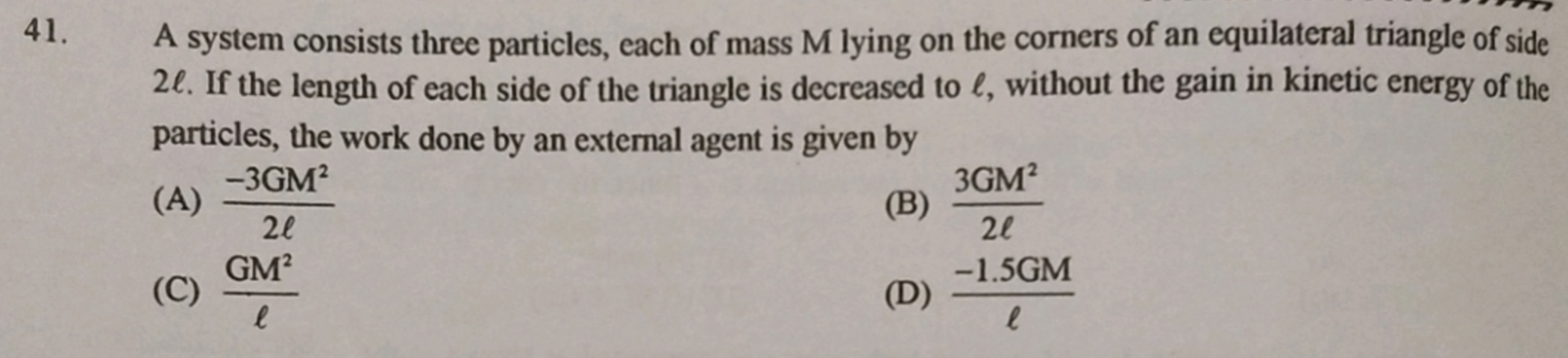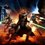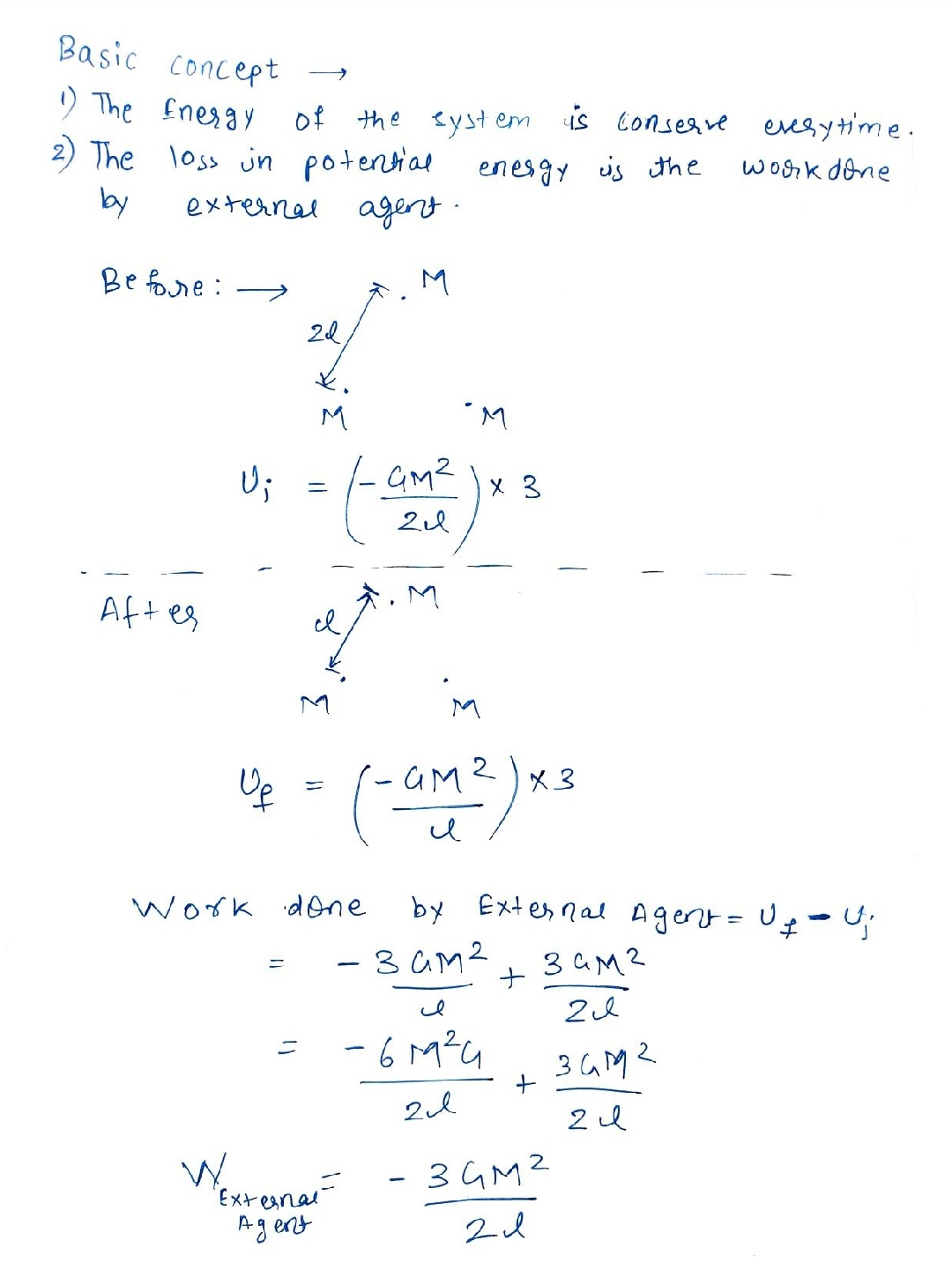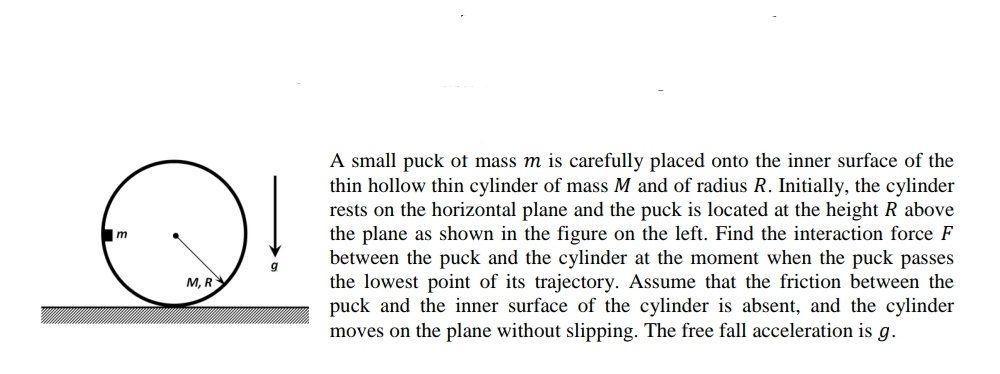# Problem no 41

3 gravitating bodies; find the work done by gravity.What answer did you get for this question? And if you don't mind, can you pls share your method?

I'm getting $\displaystyle W = -\frac{3GM^2}{2l}$;

I integrated the force by the displacement; this results in this complicated integral:

$\large \displaystyle \int_{0}^{\frac{2}{\sqrt{3}} l} \frac{\sqrt{3}gm^2}{ \left(2l - \frac{2}{\sqrt{3}}r \right)^2 } dr$

That's 'cause I totally forgot about energy-work theorem lolNote by Krishna Karthik
5 months, 4 weeks ago

This discussion board is a place to discuss our Daily Challenges and the math and science related to those challenges. Explanations are more than just a solution — they should explain the steps and thinking strategies that you used to obtain the solution. Comments should further the discussion of math and science.

When posting on Brilliant:

• Use the emojis to react to an explanation, whether you're congratulating a job well done , or just really confused .
• Ask specific questions about the challenge or the steps in somebody's explanation. Well-posed questions can add a lot to the discussion, but posting "I don't understand!" doesn't help anyone.
• Try to contribute something new to the discussion, whether it is an extension, generalization or other idea related to the challenge.

MarkdownAppears as
*italics* or _italics_ italics
**bold** or __bold__ bold
- bulleted- list
• bulleted
• list
1. numbered2. list
1. numbered
2. list
Note: you must add a full line of space before and after lists for them to show up correctly
paragraph 1paragraph 2

paragraph 1

paragraph 2

[example link](https://brilliant.org)example link
> This is a quote
This is a quote
    # I indented these lines
# 4 spaces, and now they show
# up as a code block.

print "hello world"
# I indented these lines
# 4 spaces, and now they show
# up as a code block.

print "hello world"
MathAppears as
Remember to wrap math in $$ ... $$ or $ ... $ to ensure proper formatting.
2 \times 3 $2 \times 3$
2^{34} $2^{34}$
a_{i-1} $a_{i-1}$
\frac{2}{3} $\frac{2}{3}$
\sqrt{2} $\sqrt{2}$
\sum_{i=1}^3 $\sum_{i=1}^3$
\sin \theta $\sin \theta$
\boxed{123} $\boxed{123}$

Sort by:

I just woke up.- 5 months, 4 weeks ago

Very good! Btw I solved the problem using a really complicated integral lol. I integrated the force by the displacement along the path of the objects, and thereby got the final solution.

- 5 months, 4 weeks ago

@Krishna Karthik very nice.
The formula which I have used comes from your method only. . I just have used shortcut.

- 5 months, 4 weeks ago

Yeah; I really need to familiarise myself with work energy theorem lol.

- 5 months, 4 weeks ago

Actually, this can be solved using process of elimination as well; firstly, we know that the work done must be negative, and for that there are only two options.

Secondly, we know that the $M$ term of the gravitational force must be of order two ($M^2$), therefore the only suitable option for the work must be (A).

- 5 months, 4 weeks ago

I just ran a numerical time-domain simulation and looked at the total kinetic energy at the end, assuming that no external force is trying to slow it down. And then the answer is just the negative of that, which turns out to be the first option (A).

  1 2 3 4 5 6 7 8 9 10 11 12 13 14 15 16 17 18 19 20 21 22 23 24 25 26 27 28 29 30 31 32 33 34 35 36 37 38 39 40 41 42 43 44 45 46 47 48 49 50 51 52 53 54 55 56 57 58 59 60 61 62 63 64 65 66 67 68 69 70 71 72 73 74 75 import math # Constants M = 2.0 L = math.sqrt(3.0)/2.0 G = 1.0 dt = 10.0**(-5.0) ################################### # Initialize simulation t = 0.0 y = 0.0 x = 1.0 # start on x axis at 1 distance unit away xd = 0.0 xdd = 0.0 # Run simulation while x >= 0.5: x = x + xd*dt # numerical integration xd = xd + xdd*dt x2 = -0.5*x # location of other particle y2 = math.sqrt(3.0)*x/2.0 Dx = x2 - x Dy = y2 - y D = math.hypot(Dx,Dy) ux = Dx/D uy = Dy/D F = 2.0*G*M*M/(D**2.0) # double the force for two particles Fx = F*ux # net force only in horizontal direction xdd = Fx/M t = t + dt ################################### E = 3.0*0.5*M*(xd**2.0) # total kinetic energy of three particles opt1 = -3.0*G*(M**2.0)/(2.0*L) # compare against options opt2 = 3.0*G*(M**2.0)/(2.0*L) opt3 = G*(M**2.0)/L opt4 = -3.0*G*M/(2.0*L) print dt print E print "" print opt1 print opt2 print opt3 print opt4 #>>> #1e-05 #6.92824539557 #-6.92820323028 #6.92820323028 #4.61880215352 #-3.46410161514 #>>> 

- 5 months, 4 weeks ago

Thanks! Yes; turns out I did get the correct answer. Thanks for this beautiful solution :) Computers are always brilliant.

- 5 months, 4 weeks ago

@Krishna Karthik whenever he uses the variable of problem as constant.
Like in this problem he has used $M=2.0$ it made me laugh 😂
He is a very smart guy😎.

- 5 months, 4 weeks ago

yea lol; but literally any variable you plug into values of $M , G, L$ it will give you same thing. Programmers like to be efficient😎

- 5 months, 4 weeks ago

@Krishna Karthik yeah I used this smart tricks in maths in my exam.
The way he chooses random values make me laugh.

- 5 months, 4 weeks ago

@Krishna Karthik Hii sir. Try this problem- 5 months, 3 weeks ago

Will do it soon! :)

- 5 months, 3 weeks ago

@Krishna Karthik would you like to play chess now?

- 5 months, 3 weeks ago

Oh lol I'm at school, but we're doing some dumb enrichment thing. I have no problem playing chess :)

- 5 months, 3 weeks ago

Ok, let's play 10 min game

- 5 months, 3 weeks ago

@Krishna Karthik it is not work done by gravity. It is workdone by external agent..

- 5 months, 4 weeks ago

Which is gravity, basically.

- 5 months, 4 weeks ago

@Krishna Karthik it can be other different forces also.

- 5 months, 4 weeks ago

Yeah, but why would there be a gravitational constant in the equation otherwise? Or, is it possible that a mechanical force would need the exact same amount of work to move the balls inward by half?

- 5 months, 4 weeks ago

@Krishna Karthik yes it is possible. If the balls come up with their own interacting force then they all have some velocity.

- 5 months, 4 weeks ago

Oh ok. Thanks for explaining. Bro you are expert in physics.

- 5 months, 4 weeks ago

@Neeraj Anand Badgujar @NJ STAR Ah, just for your interest, here is the integral which I calculated to solve the problem:

$\large \displaystyle \int_{0}^{\frac{2}{\sqrt{3}} l} \frac{\sqrt{3}gm^2}{ \left(2l - \frac{2}{\sqrt{3}}r \right)^2 } dr$

- 5 months, 4 weeks ago

Hey guys; I kinda need help with a problem (problem 41). I've posted it in a new note. If you can help me that would be awesome. Cheers!

- 5 months, 3 weeks ago

I need help with a new problem I'm posting; it's an error in Matlab code (coded in python style so it's easy to understand for python users too). I've posted it in a new note.

- 5 months ago